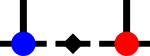SyTen## ◆ IdentityDenseTensor

template<Rank rank, typename Scalar = SDef>
 using syten::IdentityDenseTensor = typedef IdentityDenseTensorImpl::IdentityDenseTensor

Specialised identity dense tensor.

This tensor represents an identity rank-2, rank-3 or rank-4 tensor. Rank-2 tensors are standard diagonal identites. Rank-4 tensor elements are one if and only if the first two and the second two coordinates match (aabb). Rank-3 tensors optionally support an offset into the tensor, essentially shifting the third dimension by some amount. Rank-3 tensor elements are one if the first coordinate scaled by the second dimension plus the second coordinate plus the offset is equal to the third coordinate.

This type of tensor is generated during merging and fusing tensor operations in genFuse() and genSplit() if the option make_special is enabled (e.g. using gME()).